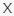# Find the Fox! Quiz VideoQuizHero

Try Version2: Find the Fox! Quiz VideoQuizHero 2

Q 1. Let’s start! Can you find the fox?

Choices:

A
B
C
D

B

Q 2. Where is the fox hiding here?

Choices:

A
B
C
D

C

Q 3. Think you can spot the fox in this picture?

Choices:

A
B
C
D

D

Q 4. Where is the fox?

Choices:

A
B
C
D

C

Q 5. Find the fox!

Choices:

A
B
C
D

B

Q 6. Look carefully. Can you find the fox?

Choices:

A
B
C
D

A

Q 7. The fox is getting better at hiding. Can you spot it?

Choices:

A
B
C
D
E
F

E

Try Version2: Find the Fox! Quiz VideoQuizHero 2

Q 8. Where is the fox here?

Choices:

A
B
C
D
E

F

Try Version2: Find the Fox! Quiz VideoQuizHero 2

Q 9. Can you spot the fox?

Choices:

A
B
C
D
E
F

F

Q 10. Where is the fox hiding here?

Choices:

A
B
C
D
E
F

A

Q 11. How many foxes can you find here? Type your answer in below!

5

9

Q 13. Count the number of foxes you see here and then type your answer in below!

13

Q 14. How many foxes are hidden here? Type your answer in below!

8

Q 15. How many foxes are hidden in this crowd? Type your answer in below!

21

Try Version2: Find the Fox! Quiz VideoQuizHero 2

### One thought on “Find the Fox! Quiz VideoQuizHero”

•April 4, 2021 at 04:45